# 7.3 Unit circle  (Page 8/11)

 Page 8 / 11

$\mathrm{sin}\text{\hspace{0.17em}}0$

## Numeric

For the following exercises, state the reference angle for the given angle.

$240°$

$60°$

$-170°$

$100°$

$80°$

$-315°$

$135°$

$45°$

$\frac{5\pi }{4}$

$\frac{2\pi }{3}$

$\frac{\pi }{3}$

$\frac{5\pi }{6}$

$\frac{-11\pi }{3}$

$\frac{\pi }{3}$

$\frac{-7\pi }{4}$

$\frac{-\pi }{8}$

$\frac{\pi }{8}$

For the following exercises, find the reference angle, the quadrant of the terminal side, and the sine and cosine of each angle. If the angle is not one of the angles on the unit circle, use a calculator and round to three decimal places.

$225°$

$300°$

$60°,$ Quadrant IV, $\text{\hspace{0.17em}}\text{sin}\left(300°\right)=-\frac{\sqrt{3}}{2},\mathrm{cos}\left(300°\right)=\frac{1}{2}$

$320°$

$135°$

$45°,$ Quadrant II, $\text{sin}\left(135°\right)=\frac{\sqrt{2}}{2},\mathrm{cos}\left(135°\right)=-\frac{\sqrt{2}}{2}$

$210°$

$120°$

Quadrant II, $\text{\hspace{0.17em}}\text{sin}\left(120°\right)=\frac{\sqrt{3}}{2},\mathrm{cos}\left(120°\right)=-\frac{1}{2}$

$250°$

$150°$

$30°,$ Quadrant II, $\text{\hspace{0.17em}}\text{sin}\left(150°\right)=\frac{1}{2},\mathrm{cos}\left(150°\right)=-\frac{\sqrt{3}}{2}$

$\frac{5\pi }{4}$

$\frac{7\pi }{6}$

$\frac{\pi }{6},$ Quadrant III, $\text{\hspace{0.17em}}\text{sin}\left(\frac{7\pi }{6}\right)=-\frac{1}{2},\mathrm{cos}\left(\frac{7\pi }{6}\right)=-\frac{\sqrt{3}}{2}$

$\frac{5\pi }{3}$

$\frac{3\pi }{4}$

$\frac{\pi }{4},$ Quadrant II, $\text{\hspace{0.17em}}\text{sin}\left(\frac{3\pi }{4}\right)=\frac{\sqrt{2}}{2},\mathrm{cos}\left(\frac{4\pi }{3}\right)=-\frac{\sqrt[]{2}}{2}$

$\frac{4\pi }{3}$

$\frac{2\pi }{3}$

$\frac{\pi }{3},$ Quadrant II, $\text{\hspace{0.17em}}\text{sin}\left(\frac{2\pi }{3}\right)=\frac{\sqrt{3}}{2},\mathrm{cos}\left(\frac{2\pi }{3}\right)=-\frac{1}{2}$

$\frac{5\pi }{6}$

$\frac{7\pi }{4}$

$\frac{\pi }{4},$ Quadrant IV, $\text{\hspace{0.17em}}\text{sin}\left(\frac{7\pi }{4}\right)=-\frac{\sqrt{2}}{2},\text{cos}\left(\frac{7\pi }{4}\right)=\frac{\sqrt{2}}{2}$

For the following exercises, find the requested value.

If $\text{\hspace{0.17em}}\text{cos}\left(t\right)=\frac{1}{7}\text{\hspace{0.17em}}$ and $\text{\hspace{0.17em}}t\text{\hspace{0.17em}}$ is in the fourth quadrant, find $\text{\hspace{0.17em}}\text{sin}\left(t\right).$

If $\text{\hspace{0.17em}}\text{cos}\left(t\right)=\frac{2}{9}\text{\hspace{0.17em}}\text{\hspace{0.17em}}$ and $\text{\hspace{0.17em}}t\text{\hspace{0.17em}}$ is in the first quadrant, find $\text{\hspace{0.17em}}\text{sin}\left(t\right).$

$\frac{\sqrt{77}}{9}$

If $\text{\hspace{0.17em}}\text{sin}\left(t\right)=\frac{3}{8}\text{\hspace{0.17em}}$ and $\text{\hspace{0.17em}}t\text{\hspace{0.17em}}$ is in the second quadrant, find $\text{\hspace{0.17em}}\text{cos}\left(t\right).$

If $\text{\hspace{0.17em}}\text{sin}\left(t\right)=-\frac{1}{4}\text{\hspace{0.17em}}$ and $\text{\hspace{0.17em}}t\text{\hspace{0.17em}}$ is in the third quadrant, find $\text{\hspace{0.17em}}\text{cos}\left(t\right).$

$-\frac{\sqrt{15}}{4}$

Find the coordinates of the point on a circle with radius 15 corresponding to an angle of $\text{\hspace{0.17em}}220°.$

Find the coordinates of the point on a circle with radius 20 corresponding to an angle of $\text{\hspace{0.17em}}120°.$

Find the coordinates of the point on a circle with radius 8 corresponding to an angle of $\text{\hspace{0.17em}}\frac{7\pi }{4}.$

Find the coordinates of the point on a circle with radius 16 corresponding to an angle of $\text{\hspace{0.17em}}\frac{5\pi }{9}.$

State the domain of the sine and cosine functions.

State the range of the sine and cosine functions.

## Graphical

For the following exercises, use the given point on the unit circle to find the value of the sine and cosine of $\text{\hspace{0.17em}}t.$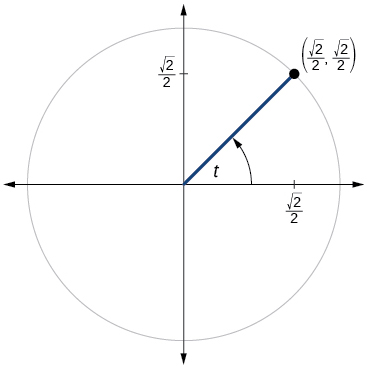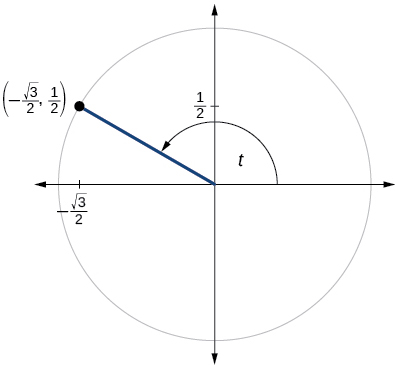$\mathrm{sin}t=\frac{1}{2},\mathrm{cos}t=-\frac{\sqrt{3}}{2}$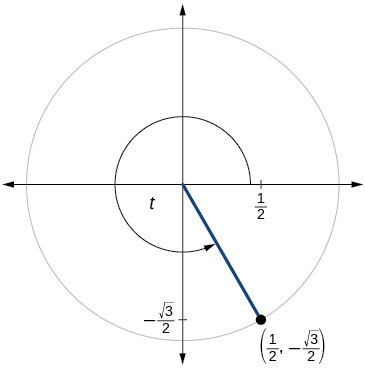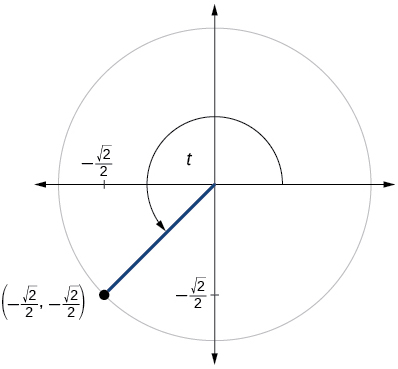$\mathrm{sin}t=-\frac{\sqrt{2}}{2},\mathrm{cos}t=-\frac{\sqrt{2}}{2}$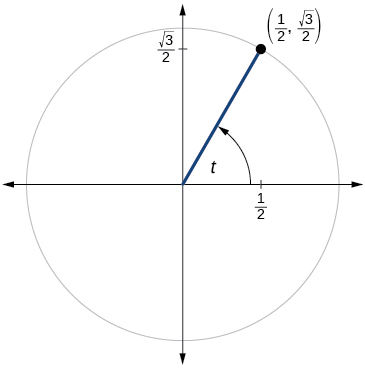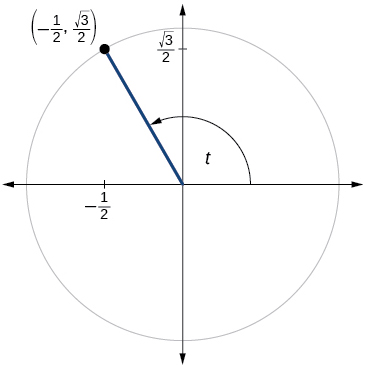$\mathrm{sin}t=\frac{\sqrt{3}}{2},\mathrm{cos}t=-\frac{1}{2}$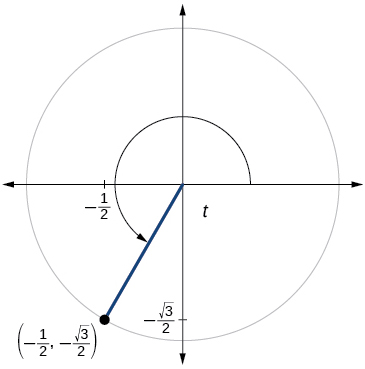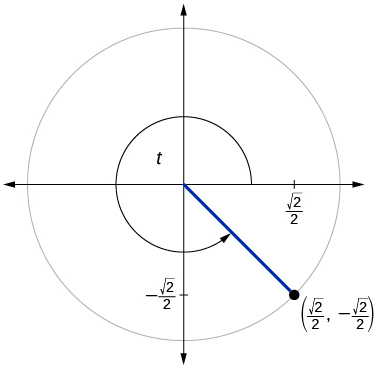$\mathrm{sin}t=-\frac{\sqrt{2}}{2},\mathrm{cos}t=\frac{\sqrt{2}}{2}$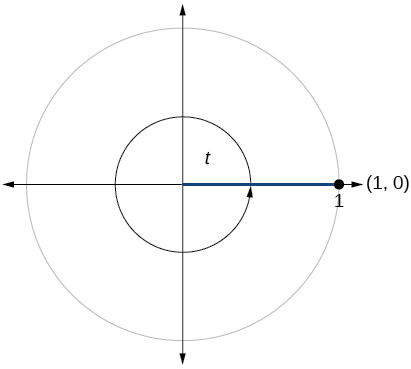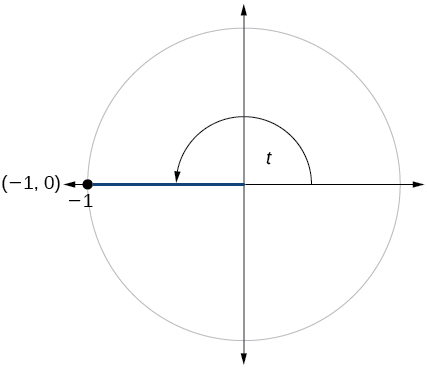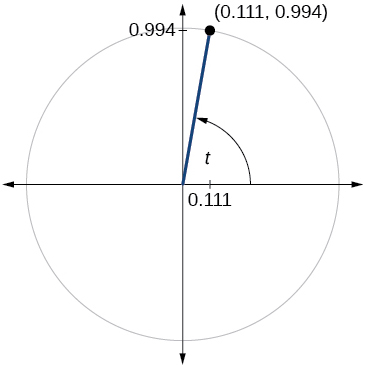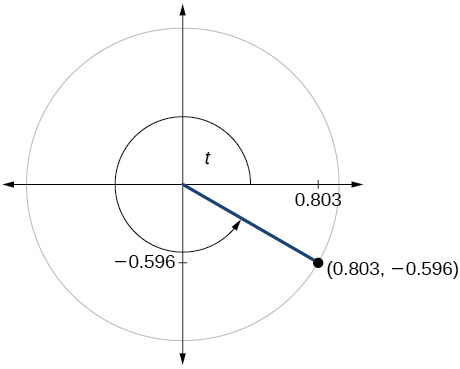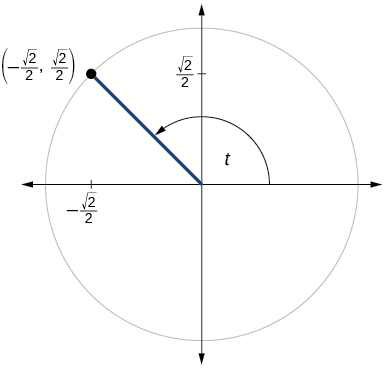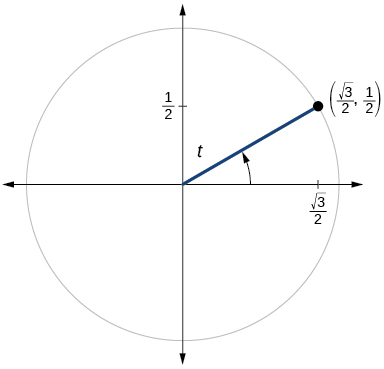$\mathrm{sin}t=\frac{1}{2},\mathrm{cos}t=\frac{\sqrt{3}}{2}$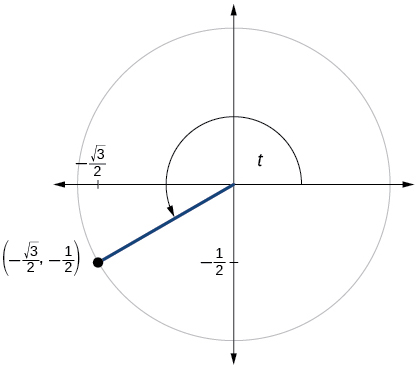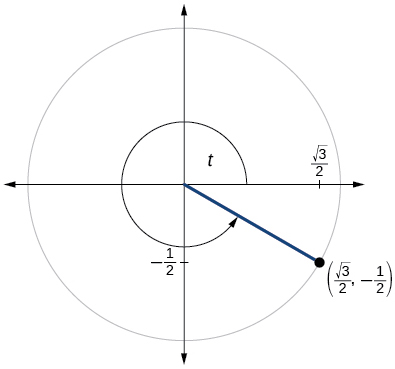$\mathrm{sin}t=-\frac{1}{2},\mathrm{cos}t=\frac{\sqrt{3}}{2}$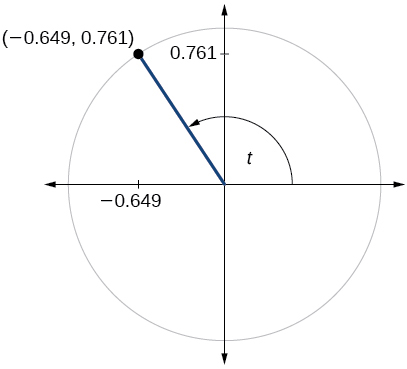$\mathrm{sin}t=0.761,\mathrm{cos}t=-0.649$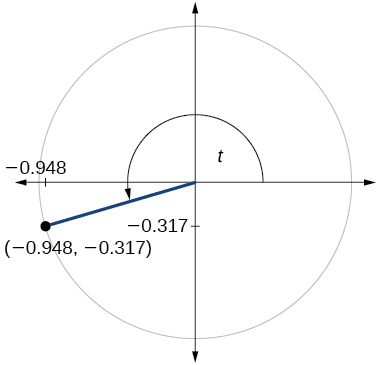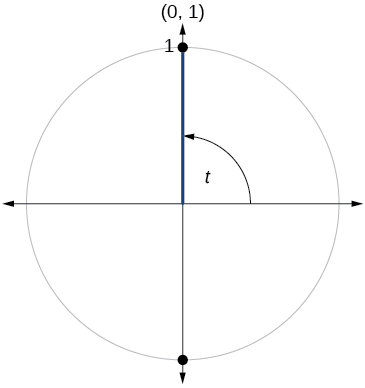$\mathrm{sin}t=1,\mathrm{cos}t=0$

## Technology

For the following exercises, use a graphing calculator to evaluate.

$\mathrm{sin}\text{\hspace{0.17em}}\frac{5\pi }{9}$

$\mathrm{cos}\text{\hspace{0.17em}}\frac{5\pi }{9}$

−0.1736

$\mathrm{sin}\text{\hspace{0.17em}}\frac{\pi }{10}$

$\mathrm{cos}\text{\hspace{0.17em}}\frac{\pi }{10}$

0.9511

$\mathrm{sin}\text{\hspace{0.17em}}\frac{3\pi }{4}$

$\mathrm{cos}\text{\hspace{0.17em}}\frac{3\pi }{4}$

−0.7071

$\mathrm{sin}\text{\hspace{0.17em}}98°$

$\mathrm{cos}\text{\hspace{0.17em}}98°$

−0.1392

$\mathrm{cos}\text{\hspace{0.17em}}310°$

$\mathrm{sin}\text{\hspace{0.17em}}310°$

−0.7660

## Extensions

For the following exercises, evaluate.

$\mathrm{sin}\left(\frac{11\pi }{3}\right)\text{\hspace{0.17em}}\mathrm{cos}\left(\frac{-5\pi }{6}\right)$

$\mathrm{sin}\left(\frac{3\pi }{4}\right)\text{\hspace{0.17em}}\mathrm{cos}\left(\frac{5\pi }{3}\right)$

$\frac{\sqrt{2}}{4}$

$\mathrm{sin}\left(-\frac{4\pi }{3}\right)\text{\hspace{0.17em}}\mathrm{cos}\left(\frac{\pi }{2}\right)$

$\mathrm{sin}\left(\frac{-9\pi }{4}\right)\text{\hspace{0.17em}}\mathrm{cos}\left(\frac{-\pi }{6}\right)$

$-\frac{\sqrt{6}}{4}$

$\mathrm{sin}\left(\frac{\pi }{6}\right)\text{\hspace{0.17em}}\mathrm{cos}\left(\frac{-\pi }{3}\right)$

$\mathrm{sin}\left(\frac{7\pi }{4}\right)\mathrm{cos}\left(\frac{-2\pi }{3}\right)$

$\frac{\sqrt{2}}{4}$

$\mathrm{cos}\left(\frac{5\pi }{6}\right)\text{\hspace{0.17em}}\mathrm{cos}\left(\frac{2\pi }{3}\right)$

$\mathrm{cos}\left(\frac{-\pi }{3}\right)\mathrm{cos}\left(\frac{\pi }{4}\right)$

$\frac{\sqrt{2}}{4}$

$\mathrm{sin}\left(\frac{-5\pi }{4}\right)\text{\hspace{0.17em}}\mathrm{sin}\left(\frac{11\pi }{6}\right)$

$\mathrm{sin}\left(\pi \right)\mathrm{sin}\left(\frac{\pi }{6}\right)$

0

## Real-world applications

For the following exercises, use this scenario: A child enters a carousel that takes one minute to revolve once around. The child enters at the point $\text{\hspace{0.17em}}\left(0,1\right),$ that is, on the due north position. Assume the carousel revolves counter clockwise.

What are the coordinates of the child after 45 seconds?

What are the coordinates of the child after 90 seconds?

$\left(0,–1\right)$

What are the coordinates of the child after 125 seconds?

When will the child have coordinates $\text{\hspace{0.17em}}\left(0.707,–0.707\right)\text{\hspace{0.17em}}$ if the ride lasts 6 minutes? (There are multiple answers.)

37.5 seconds, 97.5 seconds, 157.5 seconds, 217.5 seconds, 277.5 seconds, 337.5 seconds

When will the child have coordinates $\text{\hspace{0.17em}}\left(–0.866,–0.5\right)\text{\hspace{0.17em}}$ if the ride lasts 6 minutes?

bsc F. y algebra and trigonometry pepper 2
given that x= 3/5 find sin 3x
4
DB
remove any signs and collect terms of -2(8a-3b-c)
-16a+6b+2c
Will
Joeval
(x2-2x+8)-4(x2-3x+5)
sorry
Miranda
x²-2x+9-4x²+12x-20 -3x²+10x+11
Miranda
x²-2x+9-4x²+12x-20 -3x²+10x+11
Miranda
(X2-2X+8)-4(X2-3X+5)=0 ?
master
The anwser is imaginary number if you want to know The anwser of the expression you must arrange The expression and use quadratic formula To find the answer
master
The anwser is imaginary number if you want to know The anwser of the expression you must arrange The expression and use quadratic formula To find the answer
master
Y
master
master
Soo sorry (5±Root11* i)/3
master
Mukhtar
explain and give four example of hyperbolic function
What is the correct rational algebraic expression of the given "a fraction whose denominator is 10 more than the numerator y?
y/y+10
Mr
Find nth derivative of eax sin (bx + c).
Find area common to the parabola y2 = 4ax and x2 = 4ay.
Anurag
A rectangular garden is 25ft wide. if its area is 1125ft, what is the length of the garden
to find the length I divide the area by the wide wich means 1125ft/25ft=45
Miranda
thanks
Jhovie
What do you call a relation where each element in the domain is related to only one value in the range by some rules?
A banana.
Yaona
given 4cot thither +3=0and 0°<thither <180° use a sketch to determine the value of the following a)cos thither
what are you up to?
nothing up todat yet
Miranda
hi
jai
hello
jai
Miranda Drice
jai
aap konsi country se ho
jai
which language is that
Miranda
I am living in india
jai
good
Miranda
what is the formula for calculating algebraic
I think the formula for calculating algebraic is the statement of the equality of two expression stimulate by a set of addition, multiplication, soustraction, division, raising to a power and extraction of Root. U believe by having those in the equation you will be in measure to calculate it
Miranda
state and prove Cayley hamilton therom
hello
Propessor
hi
Miranda
the Cayley hamilton Theorem state if A is a square matrix and if f(x) is its characterics polynomial then f(x)=0 in another ways evey square matrix is a root of its chatacteristics polynomial.
Miranda
hi
jai
hi Miranda
jai
thanks
Propessor
welcome
jai
What is algebra
algebra is a branch of the mathematics to calculate expressions follow.
Miranda
Miranda Drice would you mind teaching me mathematics? I think you are really good at math. I'm not good at it. In fact I hate it. 😅😅😅
Jeffrey
lolll who told you I'm good at it
Miranda
something seems to wispher me to my ear that u are good at it. lol
Jeffrey
lolllll if you say so
Miranda
but seriously, Im really bad at math. And I hate it. But you see, I downloaded this app two months ago hoping to master it.
Jeffrey
which grade are you in though
Miranda
oh woww I understand
Miranda
Jeffrey
Jeffrey
Miranda
how come you finished in college and you don't like math though
Miranda
gotta practice, holmie
Steve
if you never use it you won't be able to appreciate it
Steve
I don't know why. But Im trying to like it.
Jeffrey
yes steve. you're right
Jeffrey
so you better
Miranda
what is the solution of the given equation?
which equation
Miranda
I dont know. lol
Jeffrey
Miranda
JeffreyByByBy OpenStaxBy OpenStaxBy Saylor FoundationBy Anh DaoBy Richley CrapoBy JavaChamp TeamBy OpenStaxBy OpenStaxBy OpenStaxBy Jesenia Wofford